# ZIO10001 - Editorial

Editorialist: Sudheera Y S

Prerequisites: Dp, trees

Problem statement:

There are a set of employees. All the employees except the head have a boss to them. You want to fire a set of people such that: if you fire an employee, you have to fire all employees below that boss ( only if there are people below the boss ). We can also fire nobody

Idea:

We will do dp on trees in this problem.

Here is our approach :

Let dp[i] store the number of ways you can fire the subtree of the ith employee

Base Case : Let us take the leaf nodes , there are two possibilities for them : either fire them or don’t fire them and they don’t have any employees below them , therefore dp[leafnodes] = 2;

Now let us see how to find the other values :

Now for any node other than leaf nodes also has two Cases : Either fire the node ( which will fire all the employees in the subtree of the node ) , or not firing the node

Case 1 : We are firing the ith node which will result in firing the all the employees in the subtree of the ith node , number of ways to do this : 1

Case 2 : We are not firing the ith node

Let us take an example and illustrate this as it is going to be easy to explain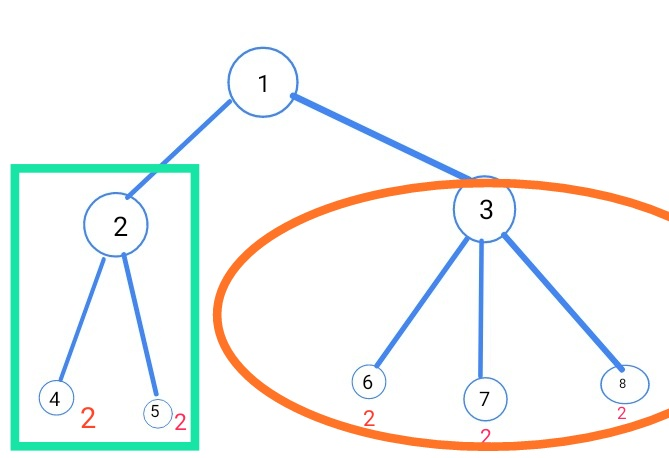In the above example nodes 4,5,6,7,8 are leaf nodes and all their dp values are 2

Now let us take 2 and find dp

Case 1: If we fire 2nd employee → number of ways 1

Case 2 : If we don’t fire the 2nd employee →

There are two ways we can fire 4 or not fire 4 and two ways of firing 5 or not firing 5

Let us make some notation

0 → means we are not firing

1 → means we are firing

4 : 0 ,1

5 : 0 ,1

Now for the 2nd employee not being fired , then we only have to consider all possibilities of 4 and 5

Which is :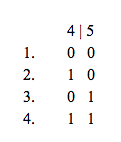Number of ways of 4 i.e dp = 2

AND

Number of ways of 5 i.e dp = 2

Total number of ways for case 2 = 2*2 = 4

NOTE : Here we can consider both 4 and 5 in dp because we have defined dp[i] as the number of ways we can fire IF WE ONLY CONSIDER SUBTREE OF i

5 doesnt come in the subtree of 4 and 4 doesn’t come in the subtree of 5 but both of them come in the subtree of 2

Therefore while calculating the dp of 2 we can consider both 4 and 5

Dp[ 2 ] = Case 1 + Case 2 = 1 + 4 = 5

Now let us calculate dp :

Case 1 : If we fire the third employee → Number of ways = 1

Case 2 : If we don’t fire the 3rd employee :

In dp , we can consider the nodes 6,7,8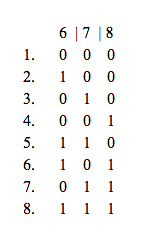Number of ways of 6 i.e dp = 2

AND

Number of ways of 7 i.e dp = 2

AND

Number of ways of 8 i.e dp = 2

Total number of ways of Case 2 : 222 = 8

Dp = Case 1 + case 2 = 1 + 8 = 9

Now let us finally find dp :

Case 1: If we fire 1st employee → Number of ways = 1

Case 2 : If we don’t fire the 1st employee :

Let us try to find the ways in which we can do 2 :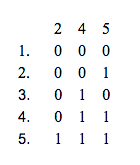Let us try to find the ways in which we can do 3 :

Now both of them ( possibilities of 2 and 3 ) are independent of each other i.e none of them comes under the same subtree , therefore Case 2 : Number of ways of 2 AND number of ways of 3 = 5*9 = 45

Dp[ 1 ] = Case 1 + Case 2 = 1 + 45 = 46

Our answer will be stored in the head of the tree because we can fire any employees and they all come under ONE subtree which is subtree of 1 therefore the answer will be stored in the head of the tree i.e dp

Here the answer is dp = 46

Now let us summarize :

Dp[i] = number of ways we can select people to fire in the SUBTREE OF i

Base case : dp[ leafnode ] = 2

For other nodes → dp[i] = dp[children] + 1

NOTE : Here is the multiplication of all the dp[children]

NOTE : If there is only 1 children for a node, then dp[node] = dp[children] + 1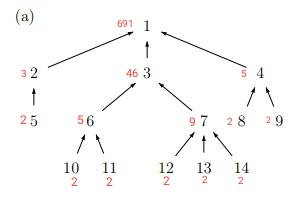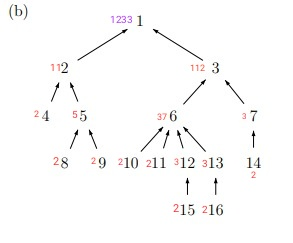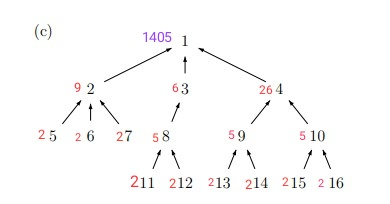Hope you understood